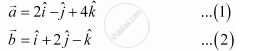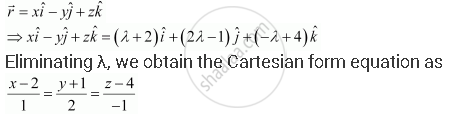Share

# Find the equation of the line in vector and in Cartesian form that passes through the point with position vector 2hati -hatj+4hatk  and is in the direction hati + 2hatj - hatk. - CBSE (Science) Class 12 - Mathematics

#### Question

Find the equation of the line in vector and in Cartesian form that passes through the point with position vector 2hati -hatj+4hatk  and is in the direction hati + 2hatj - hatk.

#### Solution

It is given that the line passes through the point with position vectorIt is known that a line through a point with position vector veca and parallel to vecb is given by the equation, vecr = veca + lambdavecb

=> vecr = 2hati - hatj +4hatk + lambda(hati+2hatj-hatk)

This is the required equation of the line in vector form.This is the required equation of the given line in Cartesian form.

Is there an error in this question or solution?

#### APPEARS IN

NCERT Solution for Mathematics Textbook for Class 12 (2018 to Current)
Chapter 11: Three Dimensional Geometry
Q: 5 | Page no. 477

#### Video TutorialsVIEW ALL 

Solution Find the equation of the line in vector and in Cartesian form that passes through the point with position vector 2hati -hatj+4hatk  and is in the direction hati + 2hatj - hatk. Concept: Equation of a Line in Space.
S